# Scale Analysis and Creation (Redux)¶

Scale Creation describes the way that many standard scales are generated from within the package. But there are other ways to create scales.

## Mode Selection¶

When one creates a scale – for example, the Pythagorean scale or a scale of the Euler-Fokker Genera – one can looks at the various modes that can be created for that scale and evaluate them by certain criteria.

The find_best_modes() function can be used for this. This function accepts and input scale, the number of tones for the mode, and the optimization functions that should be used for evaluating the scale.

As an example, one scale that’s I’ve used in compositions is created from choosing a seven-note mode from a harmonic scale, optimized over the metric sum_p_q_for_all_intervals(). This particular scale is based upon the harmonic series referenced to the fourth harmonic.

The following code:

harmonic_scale = create_harmonic_scale(4,30)
modes = find_best_modes(harmonic_scale,
num_tones=7,
sort_order = ['sum_p_q_for_all_intervals'],
num_scales=1,
metric_function = sum_p_q_for_all_intervals)


yields the following object:

[{'mask': (0, 2, 4, 5, 8, 12, 14, 15),
'original_scale': [1,
17/16,
9/8,
19/16,
5/4,
21/16,
11/8,
23/16,
3/2,
25/16,
13/8,
27/16,
7/4,
29/16,
15/8,
2],
'scale': [1, 9/8, 5/4, 21/16, 3/2, 7/4, 15/8, 2],
'steps': [2, 2, 1, 3, 4, 2, 1],
'sum_p_q_for_all_intervals': 572}]


The returned scale: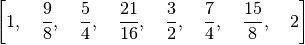minimizes the metric for all possible combinations of 7 notes chosen from the original harmonic scale.

pytuning.scale_creation.find_best_modes(scale, num_tones, sort_order=['sum_p_q_for_all_intervals', 'sum_p_q', 'sum_distinct_intervals'], num_scales=1, metric_function=None)

Find the best modes for a scale, as defined by the specified metrics.

Parameters: scale – The scale to analyze num_tones – The number of degrees in the mode sort_order – How the return should be sorted, referenced to the metrics calculated num_scales – The number of scales to return. If None all scales will be returned metric_function – The metric function to use. If None then all_metrics will be used. A sorted list of mode objects.

The sort order is a list of keys that the metric function should return, applied in order, with an assumption that the lower the metric the more consonant (and “better”) the scale. As an example, the default sort order:

['sum_p_q_for_all_intervals','sum_p_q','sum_distinct_intervals']


Will order the scales by increasing sum_p_q_for_all_intervals. If two scales have the same sum_p_q value they will be secondarily sorted on sum_p_q. If scales have the same sum_p_q_for_all_intervals and sum_p_q then sum_distinct_intervals will be used.

If no metric function is specified the default all_metrics will be used. However, for efficiency one may not want to calculate all metrics if they are not being used. For example, if one is just interested in one metric, you can pass the metric directly:

from pytuning import create_pythagorean_scale
from pytuning.metrics import sum_p_q_for_all_intervals

pythag = create_pythagorean_scale()
my_metric = lambda scale: dict(sum_p_q(scale), **sum_p_q_for_all_intervals(scale))

best_modes = find_best_modes(pythag, 7, sort_order=['sum_p_q_for_all_intervals'],
num_scales=1, metric_function=sum_p_q_for_all_intervals)


which would yield:

[{'mask': (0, 1, 3, 5, 6, 8, 10, 12),
'original_scale': [1,
256/243,
9/8,
32/27,
81/64,
4/3,
3/2,
128/81,
27/16,
16/9,
243/128,
2],
'scale': [1, 256/243, 32/27, 4/3, 1024/729, 128/81, 16/9, 2],
'steps': [1, 2, 2, 1, 2, 2, 2],
'sum_p_q_for_all_intervals': 4374}]


If one is interested in two of the metrics, you could, for example:

from pytuning import create_pythagorean_scale
from pytuning.metrics import sum_p_q, sum_p_q_for_all_intervals

pythag = create_pythagorean_scale()
my_metric = lambda scale: dict(sum_p_q(scale), **sum_p_q_for_all_intervals(scale))

best_modes = find_best_modes(pythag, 7, sort_order=['sum_p_q','sum_p_q_for_all_intervals'],
num_scales=1, metric_function=my_metric)


which would yield:

[{'mask': (0, 2, 3, 5, 7, 9, 10, 12),
'original_scale': [1,
256/243,
9/8,
32/27,
81/64,
4/3,
3/2,
128/81,
27/16,
16/9,
243/128,
2],
'scale': [1, 9/8, 32/27, 4/3, 3/2, 27/16, 16/9, 2],
'steps': [2, 1, 2, 2, 2, 1, 2],
'sum_p_q': 161,
'sum_p_q_for_all_intervals': 4374}]


## Factoring an Interval¶

Sometimes it is interesting to take an interval and find an expression for that interval over some set of generator intervals. For this the function find_factors() is provided.

One has to specify the generator intervals. This is done by passing the function a list of tuples. Each tuple has two members: The generator interval, and a character representation of the generator interval. Usually these are a single, unique character (such as X), but it can also be in the form 1/X. If it is in this form the generator interval should be the reciprocal of the interval designated by X.

As an example, we could create a generator interval that represents the tone and semi-tone of a 31-EDO scale:

edo31_constructors = [
(sp.power.Pow(2,sp.Rational(2,31)), "T"), # a tone
(sp.power.Pow(2,sp.Rational(1,31)), "s"), # a semitone
]


(Note that the tone is just twice the semitone, so we could probably get by with just defining the semitone).

Now we can define an interval, say, one of the intervals of the Pythagorean scale: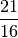and see what factoring yields an interval closest to the original.

results = find_factors(interval, edo31_constructors)
results


results now contains the factoring, the factoring in symbolic terms, and the resultant interval.

([2**(2/31), 2**(2/31), 2**(2/31), 2**(2/31), 2**(2/31), 2**(2/31)],
['T', 'T', 'T', 'T', 'T', 'T'],
2**(12/31))


The last entry is the returned interval: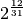If one is interested in seeing how closely the factored interval matches the original interval, the ratio_to_cents() function in pytuning.utiities can be used.

from pytuning.utilities import ratio_to_cents
print(ratio_to_cents(results / interval))


yields:

-6.26477830225428


In other words, the derived interval is flat about 6.3 cents from the target interval.

pytuning.utilities.ratio_to_cents(ratio)

Convert a scale degree to a cent value

Parameters: ratio – The scale degree (sympy value) The scale degree in cents

Calculates: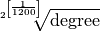Note that this function returns a floating point number, not a sympy ratio.

## Approximating a Scale with Another Scale¶

The above factoring of an interval over a set of generators can be extended: a scale can be factored too.

To do this the create_scale_from_scale() function is used.

The first step in using this function is to create an interval function. It is similar to find_factors() in that it accepts an interval and a max factor, and it returns the factor. But the actual generator intervals are bound to this function.

The easiest way of creating this function is to take the generator intervals that you’re interested in and to bind them to find_factors() via a partial function application. As an example, we can take the five-limit constructors:

five_limit_constructors = [
(sp.Rational(16,15), "s"),
(sp.Rational(10,9),  "t"),
(sp.Rational(9,8),   "T"),
]


And use them to approximate the Pythagorean scale:

from pytuning.scales import create_pythagorean_scale
from pytuning.scale_creation import create_scale_from_scale, find_factors
from pytuning.constants import five_limit_constructors
from functools import partial

interval_function = partial(find_factors, constructors=five_limit_constructors)
pythag = create_pythagorean_scale()
results = create_scale_from_scale(pythag, interval_function)


The return value is a tuple, the first element of which is derived scale, the second of which is the symbolic factoring. The scale which was found was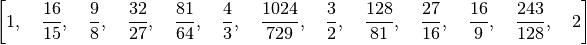If you look at the Pythagorean scale: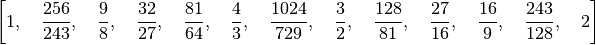you can see that they only differ in the second degree (which if we look at the first member of the return we can see is factored as [‘s’]). Looking at how much they differ:

ratio = results / pythag
print(ratio)
81/80
print(ratio_to_name(ratio))
Syntonic Comma
delta = ratio_to_cents(ratio)
print(delta)
21.5062895967149


we see that the difference is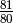, which is the syntonic comma (about 21.5 cents).

create_scale_from_scale() can also accept a tone_table which is a list of the potential breakdowns that can be used in the analysis.

pytuning.scale_creation.create_scale_from_scale(scale, interval_function, max_terms=8, tone_table=None)

Given a target scale, calculate the closest matching N-rank linear temperament scale over the provide basis functions.

Parameters: scale – The target scale (list or ratios) interval_function – The interval function (see below) max_terms – The maximum number of terms for the factoring along the basis intervals tone_table – A constrained tone table (see below) A tuple, the first member of which is a list of the derived scale values, and the second of which is a symbolic representation of the factoring found.

The interval function is a function that accepts a degree and max_terms and returns the breakdown in the same format as find_factors. In general the easiest way to create this function is thought a partial function application of find_factors and the specific constructors wanted. As an example, to create a function that will approimate a scale with the five-limit constructors:

from pytuning.scale_creation import find_factors, create_scale_from_scale
from pytuning.constants import five_limit_constructors
import functools

find_five_limit_interval = functools.partial(
find_factors, constructors=five_limit_constructors,
max_terms=15)

create_five_limit_scale_from_scale = functools.partial(create_scale_from_scale,
interval_function=find_five_limit_interval)


if tone_table is None, the code will perform the factoring with up to max_terms. If the tone table is defined only the intervals defined in this table will be used.

The tone table is formatted as a list of tuples, where the members of each tuple are the degree name (String), the interval composition (a list of characters taken from the symbolic portion of the constructors), and the value of that factoring. As an example, the tone table that matches published values for the Lucy-tuned scale begins:

[('1', [], 1),
('5', ['L', 'L', 'L', 's'], sqrt(2)*2**(1/(4*pi))),
('2', ['L'], 2**(1/(2*pi))),
('6', ['L', 'L', 'L', 'L', 's'], sqrt(2)*2**(3/(4*pi))),
('3', ['L', 'L'], 2**(1/pi)),
('7', ['L', 'L', 'L', 'L', 'L', 's'], sqrt(2)*2**(5/(4*pi))),
('#4', ['L', 'L', 'L'], 2**(3/(2*pi))), ...]


(The last member of the tuple is a sympy symbolic value.)

With the tone table, the code will return the defined tone which most closely matches the target degree.

Extending the above example, if we were to try to match a Pythagorean scale with an unconstrained factoring of the Five-limit intervals (i.e., with no tone table):

pythag = create_pythagorean_scale()
lp = create_five_limit_scale_from_scale(pythag)


yields

([1,
16/15,
9/8,
32/27,
81/64,
4/3,
3/2,
128/81,
27/16,
16/9,
243/128,
2],
[[],
['s'],
['T'],
['s', 't'],
['T', 'T'],
['T', 's', 't'],
['s', 's', 't', 't'],
['T', 'T', 's', 't'],
['T', 's', 's', 't', 't'],
['T', 'T', 'T', 's', 't'],
['T', 'T', 's', 's', 't', 't'],
['T', 'T', 'T', 'T', 's', 't'],
['T', 'T', 'T', 's', 's', 't', 't']])


Note that the first entry of the factors is always for the ratio 1, and is returned as an empty list (as there really are no factors in this sense).